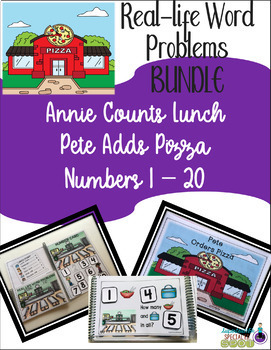# Addition Real-Life Word Problems BUNDLE 1-20Subject
Resource Type
File Type

Zip

(15 MB|30 pages)
Standards
2 Products in this Bundle
2 products
1. ANNIE ADDS THE LUNCH COUNTIt’s Annie’s day to do the lunch count in her classroom and she needs to count the lunch items and add them together so the lunchroom knows how many lunches to make for her class. This set of lunch count task cards provides your students with practice in reading a lunch cou
2. Pete Adds Pizza Orders This product is part of bundle which you will save you money when purchased together with Annie Adds Lunch Count This resource is a flexible small group or independent work station/center activity to practice interpreting information from a pizza topping order chart, using t
• Bundle Description
• StandardsNEW
Addition Real-Life Word Problems BUNDLE 1-20
This product is a bundled product of Annie Adds Lunch Count and Pete Adds Pizza: Real Life Word Problems.
Purchase these together here you save money when compared to purchasing these products separately.

This resource is a flexible small group or independent work station/center
activity to practice interpreting information from a pizza topping order chart and a lunch count menu.
Using that information to construct addition problems for numbers 1 – 20.
Students add the pizza topping or lunch count items listed on the chart.
Using task cards asking “how many” and addends and sums from 1 – 20, students work out the simple addition problems using the items and/or number cards
and record them on the task cards.

Each product has a set of 20 task cards provides the task cards in 3 differentiated (text, text + picture, and picture) to make it easy to use in your classroom. Each set is designated by different symbol in the bottom left corner of each card to facilitate sorting. Use all the cards or just some of the set to suit the needs of your students.

You also have the option of using the vertical and horizontal equation charts for
students to lay out their problems for better visualization.

WHAT YOU GET
⭐5 different Pete’s Pizza Order cards AND 5 different Annie Counts Lunch menus
⭐3 leveled sets each with 20 task cards with each set
⭐Number cards 0 – 20
⭐Picture tokens for the lunch cunt and pizza items..

STANDARDS COVERED
1. Given a number from 1-20,
as 10 things arranged in an array,
2. Given a number from 1-20, count out that many objects and add them to find the
sum.
4. Represent and solve problems involving addition.
5. Use addition to 20 to solve word problems involving situations of adding to, putting
together, and comparing, by using objects, drawings, and equations with a
symbol for the unknown number to represent the problem.
PREP FOR USE
1.Following printing, cut task cards sheets in half.
2. Cut out number cards and pizza topping cards.
3. You can laminate if you choose and add Velcro to task cards and number
cars if desired.

Related Products
Number 1-9 Numeral Cards with Touch Points
Nickel Coin Cup Matching Task Cards 5-50 Cents FREEBIE
More or Less Than Number Game {Common Core}

If at any time you have questions or concerns, please don’t hesitate to contact
me at
You can also find me at SpecialEdSpot.com
and
Fluently add and subtract within 20 using mental strategies. By end of Grade 2, know from memory all sums of two one-digit numbers.
Use addition and subtraction within 100 to solve one- and two-step word problems involving situations of adding to, taking from, putting together, taking apart, and comparing, with unknowns in all positions, e.g., by using drawings and equations with a symbol for the unknown number to represent the problem.
Determine the unknown whole number in an addition or subtraction equation relating three whole numbers. For example, determine the unknown number that makes the equation true in each of the equations 8 + ? = 11, 5 = ▯ - 3, 6 + 6 = ▯.
Understand the meaning of the equal sign, and determine if equations involving addition and subtraction are true or false. For example, which of the following equations are true and which are false? 6 = 6, 7 = 8 - 1, 5 + 2 = 2 + 5, 4 + 1 = 5 + 2.
Add and subtract within 20, demonstrating fluency for addition and subtraction within 10. Use strategies such as counting on; making ten (e.g., 8 + 6 = 8 + 2 + 4 = 10 + 4 = 14); decomposing a number leading to a ten (e.g., 13 - 4 = 13 - 3 - 1 = 10 - 1 = 9); using the relationship between addition and subtraction (e.g., knowing that 8 + 4 = 12, one knows 12 - 8 = 4); and creating equivalent but easier or known sums (e.g., adding 6 + 7 by creating the known equivalent 6 + 6 + 1 = 12 + 1 = 13).
Total Pages
30 pages
N/A
Teaching Duration
30 minutes
Report this Resource to TpT
Reported resources will be reviewed by our team. Report this resource to let us know if this resource violates TpT’s content guidelines.